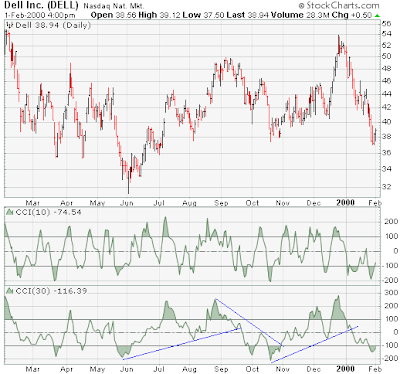CCI

Introduction
Developed by Donald Lambert, the Commodity Channel Index (CCI) was designed to identify cyclical turns in commodities. The assumption behind the indicator is that commodities (or stocks or bonds) move in cycles, with highs and lows coming at periodic intervals. Lambert recommended using 1/3 of a complete cycle (low to low or high to high) as a time frame for the CCI. (Note: Determination of the cycle's length is independent of the CCI.) If the cycle runs 60 days (a low about every 60 days), then a 20-day CCI would be recommended. For the purpose of this example, a 20-day CCI is used.

Calculation

There are 4 steps involved in the calculation of the CCI:
1. Calculate the last period's Typical Price (TP) = (H+L+C)/3 where H = high, L = low, and C = close.
2. Calculate the 20-period Simple Moving Average of the Typical Price (SMATP).
3. Calculate the Mean Deviation. First, calculate the absolute value of the difference between the last period's SMATP and the typical price for each of the past 20 periods. Add all of these absolute values together and divide by 20 to find the Mean Deviation.
4. The final step is to apply the Typical Price (TP), the Simple Moving Average of the Typical Price (SMATP), the Mean Deviation and a Constant (.015) to the following formula:
CCI = ( Typical Price - SMATP ) / ( .015 X Mean Deviation )For scaling purposes, Lambert set the constant at .015 to ensure that approximately 70 to 80 percent of CCI values would fall between -100 and +100. The CCI fluctuates above and below zero. The percentage of CCI values that fall between +100 and -100 will depend on the number of periods used. A shorter CCI will be more volatile with a smaller percentage of values between +100 and -100. Conversely, the more periods used to calculate the CCI, the higher the percentage of values between +100 and -100.

Lambert's trading guidelines for the CCI focused on movements above +100 and below -100 to generate buy and sell signals. Because about 70 to 80 percent of the CCI values are between +100 and -100, a buy or sell signal will be in force only 20 to 30 percent of the time. When the CCI moves above +100, a security is considered to be entering into a strong uptrend and a buy signal is given. The position should be closed when the CCI moves back below +100. When the CCI moves below -100, the security is considered to be in a strong downtrend and a sell signal is given. The position should be closed when the CCI moves back above -100.
Since Lambert's original guidelines, traders have also found the CCI valuable for identifying reversals. The CCI is a versatile indicator capable of producing a wide array of buy and sell signals.
• CCI can be used to identify overbought and oversold levels. A security would be deemed oversold when the CCI dips below -100 and overbought when it exceeds +100. From oversold levels, a buy signal might be given when the CCI moves back above -100. From overbought levels, a sell signal might be given when the CCI moved back below +100.
• As with most oscillators, divergences can also be applied to increase the robustness of signals. A positive divergence below -100 would increase the robustness of a signal based on a move back above -100. A negative divergence above +100 would increase the robustness of a signal based on a move back below +100.
• Trend line breaks can be used to generate signals. Trend lines can be drawn connecting the peaks and troughs. From oversold levels, an advance above -100 and trend line breakout could be considered bullish. From overbought levels, a decline below +100 and a trend line break could be considered bearish.
Traders and investors use the CCI to help identify price reversals, price extremes and trend strength. As with most indicators, the CCI should be used in conjunction with other aspects of technical analysis. CCI fits into the momentum category of oscillators. In addition to momentum, volume indicators and the price chart may also influence a technical assessment.

Example

The 20-day CCI for Brooktrout (BRKT) provides an example using Lambert's guidelines. Even though a few signals are good, using crosses above and below +100/-100 resulted in plenty of whipsaws. In January, the stock broke resistance at 20, and proceeded to double in the next few weeks. The CCI moved above and below +100 several times, but the stock remained in a strong uptrend. The CCI did manage to remain above +50 for about 7 weeks (blue oval), but the whipsaws below +100 could have caused an early exit. Whipsaws do not make an indicator bad. However, traders and investors should learn to use the CCI in conjunction with other indicators and chart analysis. In addition, various time frames for the CCI should be tested, and you should test buy and sell points, as well. What works for one stock may not necessarily work for another stock. For Brooktrout, a buy point on a cross above and below +50 may have worked better.

CCI and SharpCharts

Using SharpCharts, the CCI can be set as an indicator above or below a security's price plot. The Parameters text box to the right sets the number of periods to calculate the indicator. The default setting is 20 periods. Horizontal lines have been set at -100, 0 and +100 to help identify extremes and centerline crossovers. When the indicator moves above +100 or below -100, the portion above or below will be shaded. A number of CCI windows can be opened on any chart, and users are invited to compare different settings.